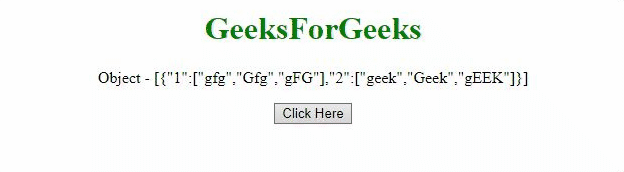# How to convert Object’s array to an array using JavaScript ?

Given an array of objects and the task is to convert the object values to an array with the help of JavaScript. There are two approaches that are discussed below:

Approach 1: We can use the map() method and return the values of each object which makes the array.

• Example:

 ` ` `<``html``> ` ` `  `<``head``> ` `    ``<``title``> ` `        ``Convert a JS object to ` `        ``an array using JQuery ` `    `` ` ` `  `    ``<``script` `src``= ` `"https://ajax.googleapis.com/ajax/libs/jquery/3.4.0/jquery.min.js"``> ` `    `` ` ` ` ` `  `<``body` `style``=``"text-align:center;"``> ` ` `  `    ``<``h1` `style``=``"color:green;"``> ` `        ``GeeksForGeeks ` `    `` ` ` `  `    ``<``p` `id``=``"GFG_UP"``> ` ` `  `    ``<``button` `onclick``=``"myGFG()"``> ` `        ``Click Here ` `    `` ` `     `  `    ``<``p` `id``=``"GFG_DOWN"``> ` ` `  `    ``<``script``> ` `        ``var up = document.getElementById("GFG_UP"); ` ` `  `        ``var JS_Obj = { ` `            ``1: ['gfg', 'Gfg', 'gFG'], ` `            ``2: ['geek', 'Geek', 'gEEK'] ` `        ``}; ` ` `  `        ``up.innerHTML = "Object - ["  ` `                ``+ JSON.stringify(JS_Obj) + "]"; ` `         `  `        ``var down = document.getElementById("GFG_DOWN"); ` `         `  `        ``function myGFG() { ` `            ``var array = \$.map(JS_Obj, function (val, ind) { ` `                ``return [val]; ` `            ``}); ` `            ``down.innerHTML = array; ` `        ``} ` `    `` ` ` ` ` `  ` `

• Output:Approach 2: The Object.keys() method is used to get the keys of object and then those keys are used to get the values of the objects from the array.

• Example:

 ` ` `<``html``> ` ` `  `<``head``> ` `    ``<``title``> ` `        ``Convert a JS object to ` `        ``an array using JQuery ` `    `` ` ` `  `    ``<``script` `src``= ` `"https://ajax.googleapis.com/ajax/libs/jquery/3.4.0/jquery.min.js"``> ` `    `` ` ` ` ` `  `<``body` `style``=``"text-align:center;"``> ` ` `  `    ``<``h1` `style``=``"color:green;"``> ` `        ``GeeksForGeeks ` `    `` ` `     `  `    ``<``p` `id``=``"GFG_UP"``> ` ` `  `    ``<``button` `onclick``=``"myGFG()"``> ` `        ``Click Here ` `    `` ` `    ``<``p` `id``=``"GFG_DOWN"``> ` ` `  `    ``<``script``> ` `        ``var up = document.getElementById("GFG_UP"); ` `         `  `        ``var JS_Obj = { ` `            ``1: ['gfg', 'Gfg', 'gFG'], ` `            ``2: ['geek', 'Geek', 'gEEK'] ` `        ``}; ` `         `  `        ``up.innerHTML = "Object - ["  ` `            ``+ JSON.stringify(JS_Obj) + "]"; ` ` `  `        ``var down = document.getElementById("GFG_DOWN"); ` `         `  `        ``function myGFG() { ` `            ``var arr = Object.keys(JS_Obj) ` `                ``.map(function (key)  ` `                ``{ return JS_Obj[key]; }); ` `                 `  `            ``down.innerHTML = arr; ` `        ``} ` `    `` ` ` ` ` `  ` `

• Output:My Personal Notes arrow_drop_upCheck out this Author's contributed articles.

If you like GeeksforGeeks and would like to contribute, you can also write an article using contribute.geeksforgeeks.org or mail your article to contribute@geeksforgeeks.org. See your article appearing on the GeeksforGeeks main page and help other Geeks.

Please Improve this article if you find anything incorrect by clicking on the "Improve Article" button below.

Practice Tags :

Be the First to upvote.

Please write to us at contribute@geeksforgeeks.org to report any issue with the above content.